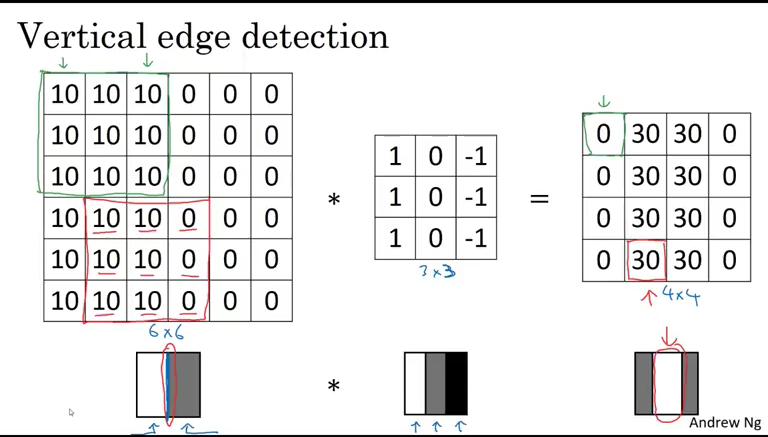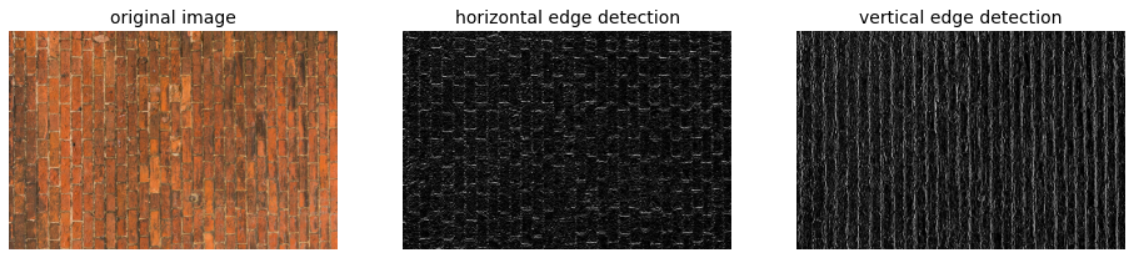# Filters in Convolutional Neural Networks

Friday, December 14, 2018

Note: This post is inspired by the answer I gave on stackoverflow.

In Convolutional Neural Networks, Filters detect spatial patterns such as edges in an image by detecting the changes in intensity values of the image.In terms of an image, a high-frequency image is the one where the intensity of the pixels changes by a large amount, whereas a low-frequency image is the one where the intensity is almost uniform. Usually, an image has both high and low frequency components. The high-frequency components correspond to the edges of an object because at the edges the rate of change of intensity of pixel values is high.

High pass filters are used to enhance the high-frequency parts of an image.

Let’s take an example that a part of your image has pixel values as [[10, 10, 0], [10, 10, 0], [10, 10, 0]] indicating the image pixel values are decreasing toward the right i.e. the image changes from light at the left to dark at the right. The filter used here is [[1, 0, -1], [1, 0, -1], [1, 0, -1]].

Now, we take the convolutional of these two matrices that give the output [[10, 0, 0], [10, 0, 0], [10, 0, 0]]. Finally, these values are summed up to give a pixel value of 30, which gives the variation in pixel values as we move from left to right. Similarly, we find the subsequent pixel values.Here, you will notice that a rate of change of pixel values varies a lot from left to right thus a vertical edge has been detected. Had you used the filter [[1, 1, 1], [0, 0, 0], [-1, -1, -1]], you would get the convolutional output consisting of 0s only i.e. no horizontal edge present. In the similar ways, [[-1, 1], [-1, 1]] detects a vertical edge.

Usually, a vertical edge detection filter has bright pixels on the left and dark pixels on the right (or vice-versa). Also, the sum of values of the filter should be 0 else the resultant image will become brighter or darker.

## ImplementationOne good example of edge detection filter is Sobel filter. Let’s implement that for edge detection.Note: In convolutional neural networks, the filters are learned the same way as hyperparameters through backpropagation during the training process.

References: